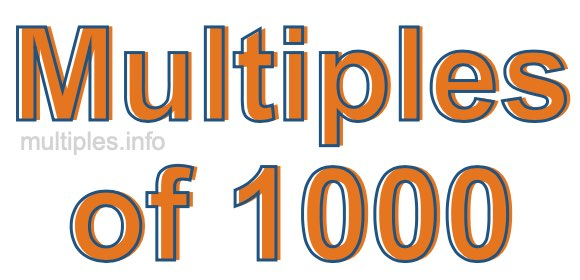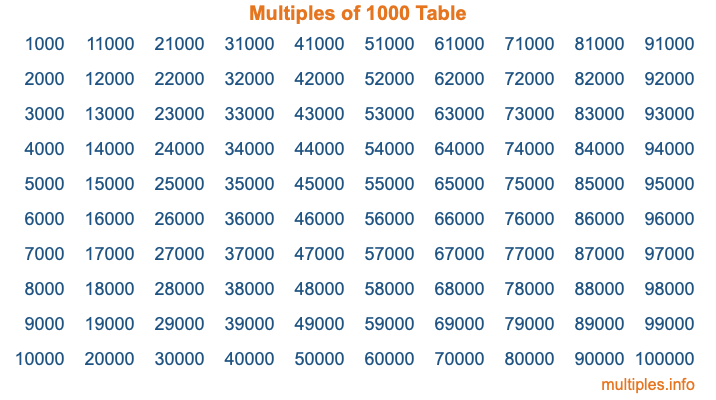Multiples of 1000Welcome to the Multiples of 1000 page. Here we will first teach you everything you will ever need to know about the multiples of 1000, and then give you a study guide summary of everything we taught you to make sure you remember it all. Use this page to look up facts and learn information about the multiples of 1000. This page will make you a multiples of one thousand expert!

Definition of Multiples of 1000
Multiples of 1000 are all the numbers that when divided by 1000 equal an integer. Each of the multiples of 1000 are called a multiple. A multiple of 1000 is created by multiplying 1000 by an integer.

Therefore, to create a list of multiples of 1000, you start with 1 multiplied by 1000, then 2 multiplied by 1000, then 3 multiplied by 1000, and so on for as long as you want. Thus, the list of the first five multiples of 1000 is 1000, 2000, 3000, 4000, and 5000. To see a larger list of multiples of 1000, see the printable image of Multiples of 1000 further down on this page. We also have a category where you can choose any nth multiple of 1000.

Multiples of 1000 Checker
The Multiples of 1000 Checker below checks to see if any number of your choice is a multiple of 1000. In other words, it checks to see if there is any number (integer) that when multiplied by 1000 will equal your number. To do that, we divide your number by 1000. If the the quotient is an integer, then your number is a multiple of 1000.

Is  a multiple of 1000?

Least Common Multiple of 1000 and ...
A Least Common Multiple (LCM) is the lowest multiple that two or more numbers have in common. This is also called the smallest common multiple or lowest common multiple and is useful to know when you are adding our subtracting fractions. Enter one or more numbers below (1000 is already entered) to find the LCM.

Check out our LCM Calculator if you need more details about the Least Common Multiple or if you need the LCM for different numbers for adding and subtraction fractions.

nth Multiple of 1000
As we stated above, 1000 is the first multiple of 1000, 2000 is the second multiple of 1000, 3000 is the third multiple of 1000, and so on. Enter a number below to find the nth multiple of 1000.

th multiple of 1000

Multiples of 1000 vs Factors of 1000
1000 is a multiple of 1000 and a factor of 1000, but that is where the similarities end. All postive multiples of 1000 are 1000 or greater than 1000. All positive factors of 1000 are 1000 or less than 1000.

Below is the beginning list of multiples of 1000 and the factors of 1000 so you can compare:

Multiples of 1000: 1000, 2000, 3000, 4000, 5000, etc.

Factors of 1000: 1, 2, 4, 5, 8, 10, 20, 25, 40, 50, 100, 125, 200, 250, 500, 1000

As you can see, the multiples of 1000 are all the numbers that you can divide by 1000 to get a whole number. The factors of 1000, on the other hand, are all the whole numbers that you can multiply by another whole number to get 1000.

It's also interesting to note that if a number (x) is a factor of 1000, then 1000 will also be a multiple of that number (x).

Multiples of 1000 vs Divisors of 1000
The divisors of 1000 are all the integers that 1000 can be divided by evenly. Below is a list of the divisors of 1000.

Divisors of 1000: 1, 2, 4, 5, 8, 10, 20, 25, 40, 50, 100, 125, 200, 250, 500, 1000

The interesting thing to note here is that if you take any multiple of 1000 and divide it by a divisor of 1000, you will see that the quotient is an integer.

Multiples of 1000 Table
Below is an image of the first 100 multiples of 1000 in a table. The table is in chronological order, column by column. The first column has the first ten multiples of 1000, the second column has the next ten multiples of 1000, and so on.The Multiples of 1000 Table is also referred to as the 1000 Times Table or Times Table of 1000. You are welcome to print out our table for your studies.

Negative Multiples of 1000
Although not often discussed or needed in math, it is worth mentioning that you can make a list of negative multiples of 1000 by multiplying 1000 by -1, then by -2, then by -3, and so on, to get the following list of negative multiples of 1000:

-1000, -2000, -3000, -4000, -5000, etc.

Multiples of 1000 Summary
Below is a summary of important Multiples of 1000 facts that we have discussed on this page. To retain the knowledge on this page, we recommend that you read through the summary and explain to yourself or a study partner why they hold true.

There are an infinite number of multiples of 1000.

A multiple of 1000 divided by 1000 will equal a whole number.

1000 divided by a factor of 1000 equals a divisor of 1000.

The nth multiple of 1000 is n times 1000.

The largest factor of 1000 is equal to the first positive multiple of 1000.

1000 is a multiple of every factor of 1000.

1000 is a multiple of 1000.

A multiple of 1000 divided by a divisor of 1000 equals an integer.

1000 divided by a divisor of 1000 equals a factor of 1000.

Any integer times 1000 will equal a multiple of 1000.

Multiples of a Number
Here you can get the multiples of another number, all with the same attention to detail as we did for multiples of 1000 on this page.

Multiples of
Multiples of 1001
Did you find our page about multiples of one thousand educational? Do you want more knowledge? Check out the multiples of the next number on our list!

Copyright  |   Privacy Policy  |   Disclaimer  |   Contact